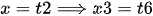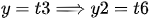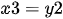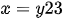## Eliminate the parameter to find a Cartesian equation of the curve. A. x = t^2 – 3, y = t + 2

Question

### Question:

Eliminate the parameter to find a Cartesian equation of the curve. A. x = t^2 – 3, y = t + 2